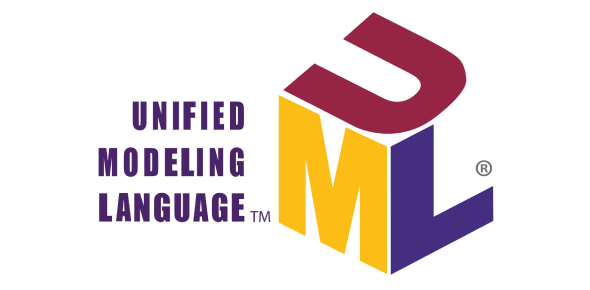# Unified Modeling Language UML Quiz

21 Questions | Total Attempts: 9086SettingsHey, check out this amazing 'Unified modeling language' quiz that is designed to test your understanding of this subject. Unified modeling language (also termed as 'UML') is a general-purpose modeling language that has proven to be a beneficial tool for software developers over the years. Take the quiz below and see how well you can score. Remember that if you score below 70%, you need to learn more about this programming language.

• 1.
What is a purpose of a Use Case diagram?
• A.

Functional decomposition

• B.

Identification of functional requirements

• C.

To define sequences of actions

• D.

To identify multiplicities

• 2.
What are the stages of the Unified Process?
• A.

Inception, Elaboration, Construction, Transition

• B.

Inception, Construction, Elaboration, Transition

• C.

Analysis, Elaboration, Testing, Transition

• D.

Design, Inception, Construction, Deployment

• 3.
Why is UML used?
• A.

Because it is a graphical language that can be used to model object oriented systems

• B.

Because it provides the software development community with a common language

• C.

Because it is similar to Java

• D.

All of the above

• 4.
In order to represent a complicated process you would use?
• A.

A Class Diagram

• B.

A Use Case diagram

• C.

An Activity Diagram

• D.

A Venn Diagram

• 5.
Construction iterations are based on?
• A.

System Activities

• B.

System Use Cases

• C.

System Classes

• D.

System States

• 6.
Stakeholders are?
• A.

Those who pay for a system

• B.

All those who specify and influence requirements

• C.

Those who will work with the system

• D.

Those who will work on the system

• 7.
Once classes and attributes have been identified and placed into a diagram, the next stage is to add?
• A.

Associations

• B.

Operations

• C.

Multiplicities

• D.

Actors

• 8.
Which is the longest phase of the Unified Process?
• A.

Construction

• B.

Transition

• C.

Inception

• D.

Elaboration

• 9.
At which stage are conceptual class diagrams used?
• A.

Construction

• B.

Transition

• C.

Inception

• D.

Elaboration

• 10.
Is the above diagram.......
• A.

A class diagram

• B.

A sequence diagram

• C.

An activity diagram

• D.

A package diagram

• 11.
For the diagram shown above, the diamond shapes represent?
• A.

A decision point

• B.

A fork node

• C.

A join node

• D.

An operation

• 12.
For the above diagram, which statement is true?
• A.

B,C,D and E will all be executed

• B.

B,D and E or C,D and E will all be executed

• C.

C,D and E will all be executed

• D.

Either C or B, D and E will be executed

• 13.
For the above diagram, which statement is true?
• A.

D and E will be executed concurrently

• B.

D and E will be executed sequentially

• C.

D and E will be executed conditionally

• D.

All the above

• 14.
For the above diagram swimlanes could be added. These would be added to......
• A.

Allow the behaviour of different objects to be shown

• B.

Allow Use Cases to be included

• C.

Allow printing of multiple page diagrams`

• D.

Allow complex systems to be analysed

• 15.
Which of the following is a valid Class name?
• A.

Ticket

• B.

Ticket sales

• C.

Ticket price

• D.

John's ticket

• 16.
Operations are used in a...........
• A.

Package diagram

• B.

Class diagram

• C.

Activity diagram

• D.

Use Case diagram

• 17.
Which of the following is a valid Use Case?
• A.

Room Number

• B.

Reserve Room

• C.

Room

• D.

Booking System

• 18.
For a restaurant receptionist identifiy the most valid Use Case.......
• A.

Eat meal

• B.

• C.

Take booking

• D.

Provide ID

• 19.
Class diagrams are used to model.......
• A.

Domains

• B.

Sequences of activity

• C.

Functional requirements

• D.

Algorithms

• 20.
A series of actions for a particular Use Case without class behaviours can be modelled using......
• A.

A Class Diagram

• B.

A Package Diagram

• C.

A Sequence Diagram

• D.

An Activity Diagram

• 21.
What is the correct order of stages of system analysis using the waterfall method?
• A.

Design, Analysis, Implementation, Deployment, Testing

• B.

Analysis, Implementation, Design, Testing, Deployment

• C.

Analysis, Design, Implementation, Testing, Deployment

• D.

Design, Analysis, Implementation, Deployment, Testing

Related Topics